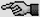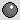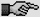# What is Boyle's gas law

##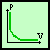The Boyle-Mariotte gas law

Page - 9 -

II. The gas laws

Derivation:
Robert Bolye carried out experiments with a U-shaped glass tube. This was sealed at one end and open at the other. He filled it with mercury (Hg) so that a small volume of air was left in the sealed tube: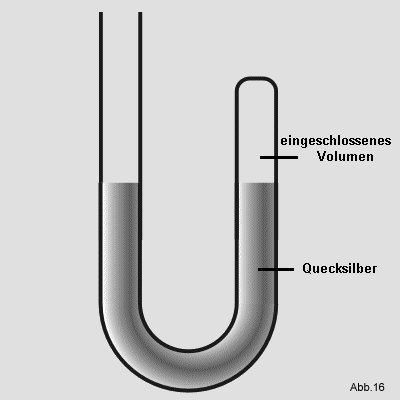He poured more mercury into the open end. The trapped air was thereby exposed to a higher pressure and the volume decreased. By measuring the pressure and the decrease in volume, Boyle found that the volume halved when the pressure was doubled.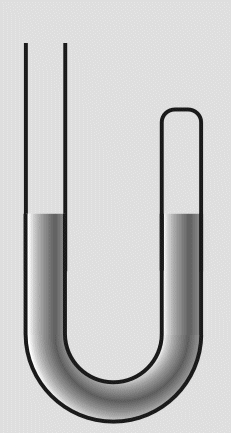Fig.17

This relationship is proportional, i.e. regardless of the value by which the pressure is changed, the volume always changes in the same ratio. This means that pressure and volume are indirectly proportional, because the volume becomes smaller with higher pressure and larger with lower pressure. This can be represented by the following equation:(8)

spelled differently:(9)

It should be noted that the temperature in Boyle's experiments was always the same.
If you enter the volume and pressure values ​​in a coordinate system, you get the following graph: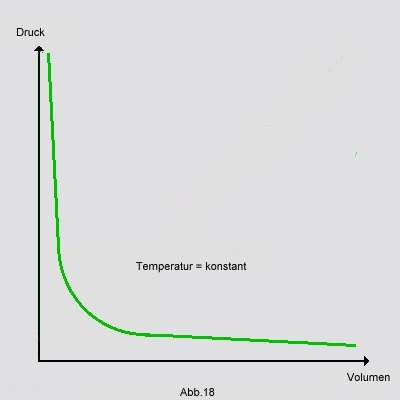If you have several related volume / pressure value pairs, you can always set their products to be the same, since the result is always constant: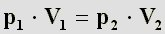(10)

The French Edm Mariotte found the same connection a few years later, hence the name, Boyle-Mariott's gas law.

Example:
You have a balloon filled with an ideal gas: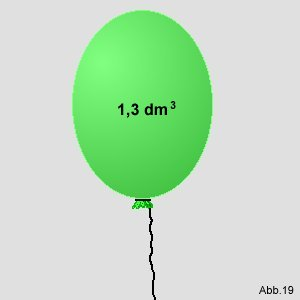Given: Its volume is 1.3 dm3. The air pressure outside the balloon is 1013 mbar (millibars).
The rubber envelope of the balloon is stretchable, but with a volume of 1.78 dm3 burst.

Question: Up to what air pressure can it rise before it bursts?

Solution: Since the temperature does not change, one can apply Boyle-Mariotte's ideal gas law. It reads as follows:This can also be rewritten by putting the two variables on one page:Since all pressure / volume value pairs always result in the same constant value, they can be equated. In this case there are only two pressure / volume value pairs, which are now equated:The given values ​​are now inserted into this equation and, according to p2 dissolved: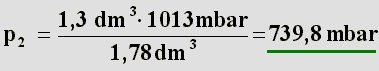Answer: The balloon bursts at an air pressure of 739.8 mbar.

 next chapter